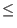Next: Parallelizing Methodology Up: A Simulation of Shor's Previous: Introduction to the Code   Contents

## The Simulation of Shor's Algorithm

The implementation of Shor's algorithm found in shor.C follows the steps outlined in the description of Shor's algorithm found above. There are some significant differences in the behavior of the simulator and the behavior of a actual quantum computer.

The simulator uses 2n+1 double precision floating point numbers to represent the state of the quantum register. It uses one Complex object to represent the probability amplitude of each of the 2n eigenstates of a n bit quantum memory register, and each Complex object uses two double precision floating point numbers. A real quantum computer would use exactly n qubits as its memory register.

A second large difference is that during the modular exponentiation step of Shor's algorithm (Step 6 above) a quantum computer would perform one operation of xa mod n, where a is the superposition of states in register 1. The simulation must calculate the superposition of values caused by calculating xa mod n for a = 0 through q - 1 iteratively. The simulation also stores the result of each modular exponentiation, and uses that information to collapse register 1 in step 7 in Shor's algorithm. A quantum computer would not be capable of performing this book keeping, as examining any particular result would collapse the existing superposition to be placed in register 2 at the end of step 6 of Shor's algorithm. A quantum computer would have no need whatsoever to do such book keeping, as when register 2 is measured in step 7, the collapse of register 1 is an automatic and unavoidable consequence of the measurement. A analogous argument follows for the use of the get probability function in step 8 of Shor's algorithm for calculating the discrete Fourier transform.

Aside from these differences, which are necessitated by the inability of a classical computer to accurately depict the behavior of a quantum mechanical system, the operations are performed by the shor.C program are identical to those called for in the description of Shor's algorithm.

As the algorithm runs the state of the quantum memory register changes in the manner laid out in the description of Shor's algorithm. After the final measurement of register 1 in step 9 we obtain some integer m, which has a high probability of being an integer multipleof q/r. r is the period of xa mod n that we are trying to find, so that we may calculate xr/2 -1 mod n and xr/2 +1 mod n in an effort to find numbers which share factors with n.

In the post processing step shor.C takes the integer m and divides it by q, thus yielding some number c which is approximately equal to/r, whereis an integer, and r is the desired period. Then using a helper function it calculates the best rational approximation to c which has a denominator that is less than or equal to q (recall that q is the power of two such that n2q < 2n2, where n is the number to be factored by Shor's algorithm). The period must be less than or equal to q, because there are only q values total, so for them to be periodic, the period must be less than q.

We take this denominator to be the desired period. Shor's algorithm can only use even periods in determining factors of n, and so we check to see if r is even, if not we check to see if doubling the period would still yield a period less than q, if so we double the guessed period.

Taking the period, which is now guaranteed to be even, we proceed to calculate xr/2 -1 mod n and xr/2 +1 mod n, calling these values a and b, we compute the greatest common denominator of a and n, and b and n, to see if we have attained a nontrivial factor of n.Next: Parallelizing Methodology Up: A Simulation of Shor's Previous: Introduction to the Code   Contents
Matthew Hayward - Quantum Computing, Shor's Algorithm, and Parallelism GitHub Repository